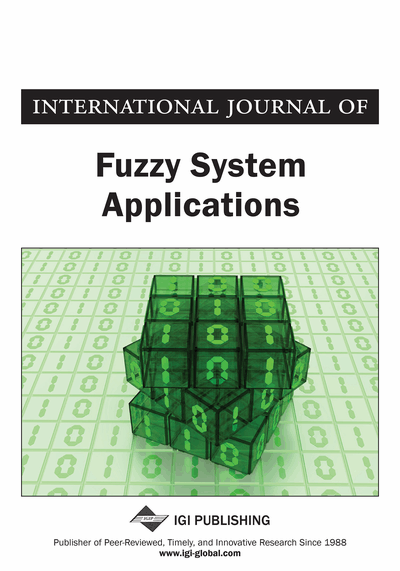# Generalized Intuitionistic Fuzzy Entropy Measure of Order α and Degree β and Its Applications to Multi-Criteria Decision Making Problem

Harish Garg, Nikunj Agarwal, Alka Tripathi
DOI: 10.4018/IJFSA.2017010105
OnDemand:
(Individual Articles)
Available
\$37.50
No Current Special Offers

## Abstract

The objective of this manuscript is divided into two fold. Firstly, a more generalized intuitionistic fuzzy entropy measure of order and degree has been presented for measuring the degree of fuzziness of the set with a proof of its validity. A structured linguistic variable has been taken as an illustrative example to show its validity and superiority than the existing measures. Furthermore, based on this measure, an approach to deal with multi-criteria decision making (MCDM) problem is developed. Finally, a practical example is provided to illustrate the decision making process. A computed result is compared with the help of existing results. A sensitivity analysis on the different values of the parameters will make a decision maker more choice for accessing their results.
Article Preview
Top

## 1. Introduction

Classical information theory has been widely used in the literature for representing the uncertainties in the data in the form of classical measure theory. But these measures are valid only for a precise data i.e., where the data related to the system are precisely known. But due to the various constraints in day-today life, decision makers may give their judgements under the uncertain and imprecise in nature. Thus, there is always a degree of hesitancy between the preferences of the decision making and hence, the analysis conducted under such circumstances are not ideal and hence does not tell the exact information to the system analyst. To handle this, fuzzy set theory (Zadeh, 1965) has been introduced for handling the uncertainties in the data by defining their membership grades between 0 and 1 corresponding to each element in the universe of discourse. Under these environments, Deluca and Termini (1971) proposed a set of axioms for the fuzzy entropy based on the Shannon measure (Shanon, 1948). After their pioneer work, various authors (Bhandari and Pal, 1993; Garg et al. 2015; Pal and Pal, 1999; Verma and Sharma, 2011) introduced the entropy measure under the fuzzy environment. But, with the growing complexities of the systems day-by-day, it is not possible to give a preference towards the alternative under the different attribute in terms of a single or exact number. Hence, to deal with it, intuitionistic fuzzy set (IFS) theory (Attanassov, 1986) is one of the most permissible theories to handle the uncertainties and impreciseness in the data than the crisp or probability theory. For this, Szmidt and Kacprzyk (2001) extended the axioms of Deluca and Termini (1971) to IFS environment and defined their corresponding axioms. Later on, corresponding to Deluca and Termini (1971) fuzzy entropy measure, Vlachos and Sergiadis (2007) extended their measure in the IFS environment. Zhang and Jiang (2008) presented a measure of intuitionistic fuzzy entropy based on a generalization of measure of Deluca and Termini (1971). Ye (2010) proposed a two entropy measure by extending the work as defined by Parkash et al. (2008). Wei et al. (2012) gives a simplified form of the Ye (2010) entropy. Verma and Sharma (2013) proposed an exponential order entropy under IFS environment. Apart from them, the various authors have addressed the problem of MCDM by using the different aggregation as well as an entropy measure based operators (Garg, 2015, 2016, 2016a,b; Garg et al., 2015; Verma and Sharma, 2014b; Xu, 2007, 2010; Zhang et al., 2013, Wan et al., 2016; Wan and Li, 2013, 2014, 2015; Wan and Dong, 2015; Wang et al. 2015, John and Henry, 2015; Nan et al. 2016; Emdjed et al. 2016, He and He 2016, He et al., 2014, 2015, 2015a). Thus, it has been concluded that the distance or entropy measures are of key importance in a number of theoretical and applied statistical inference and data processing problems.

## Complete Article List

Search this Journal:
Reset
Volume 12: 1 Issue (2023)
Volume 11: 4 Issues (2022)
Volume 10: 4 Issues (2021)
Volume 9: 4 Issues (2020)
Volume 8: 4 Issues (2019)
Volume 7: 4 Issues (2018)
Volume 6: 4 Issues (2017)
Volume 5: 4 Issues (2016)
Volume 4: 4 Issues (2015)
Volume 3: 4 Issues (2013)
Volume 2: 4 Issues (2012)
Volume 1: 4 Issues (2011)
View Complete Journal Contents Listing